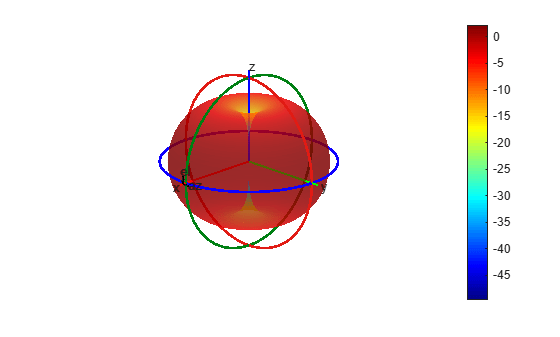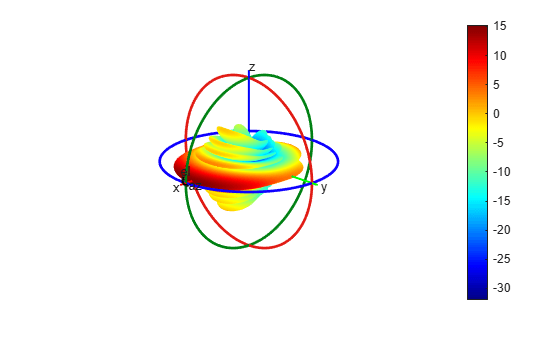# patternFromSlices

Reconstruct approximate 3-D radiation pattern from two orthogonal slices

## Syntax

``patternFromSlices(vertislice,theta,horizslice,phi)``
``patternFromSlices(vertislice,theta,horizslice)``
``patternFromSlices(vertislice,theta)``
``[pat3D,thetaout,phiout] = patternFromSlices(___)``
``[___] = patternFromSlices(___,Name,Value)``

## Description

example

````patternFromSlices(vertislice,theta,horizslice,phi)` plots the approximate 3-D pattern reconstructed from the input data containing the 2-D pattern along the vertical and horizontal plane as well as the polar and azimuthal angles in the spherical coordinates.```

example

````patternFromSlices(vertislice,theta,horizslice)` plots the approximate 3-D pattern with the horizontal slice provided as a real-valued scalar. The syntax assumes that the antenna is omnidirectional with symmetry about the Z-axis.```
````patternFromSlices(vertislice,theta)` plots the approximate 3-D pattern reconstructed from only vertical pattern data, along with the assumption of azimuthal omni directionality and that horizontal pattern data is equal to maximum value of vertical pattern data.```
````[pat3D,thetaout,phiout] = patternFromSlices(___)` returns the reconstructed pattern as a matrix with the vectors of phi and theta.```
````[___] = patternFromSlices(___,Name,Value)` provides a way to specify customization and tuning options to the pattern reconstruction method.```

## Examples

collapse all

Load the `MAT` file containing the data of the dipole pattern.

`load dipoleAntennaSlices.mat`

Reconstruct the pattern from the data provided using the `CrossWeighted `method.

`patternFromSlices(vertSlice,theta,horizSlice,phi,'Method','CrossWeighted')`Load the MAT file containing the data of the sector antenna pattern.

`load sectorAntennaSlices.mat`

Reconstruct the pattern from the data provided using the `Summing `method.

` patternFromSlices(vertSlice,theta,horizSlice,phi,'Method','Summing')```` [pat3D,thetaout,phiout] = patternFromSlices(vertSlice,theta,horizSlice,phi,'Method','Summing'); pat3D = pat3D(1:5)```
```pat3D = 1×5 -23.2025 -23.2071 -23.2224 -23.2485 -23.2854 ```
` thetaout = thetaout(1:5)`
```thetaout = 1×5 180 179 178 177 176 ```
` phiout = phiout(1:5)`
```phiout = 1×5 -180 -179 -178 -177 -176 ```

## Input Arguments

collapse all

#### Required Input Arguments

2-D pattern slice data along the vertical or the elevation plane, specified as a real-valued vector with each element unit in dBi. This parameter need not be normalized. The `numel(vertislice)` must be equal to `numel(theta)`.

Data Types: `double`

Polar or inclination angles in spherical coordinates, specified as a real-valued vector with each element unit in degrees.

### Noteel is the elevation angle.

Example: 70e6

Data Types: `double`

#### Optional Input Arguments

2-D pattern slice data along the horizontal or the azimuthal plane, specified as a real-valued scalar in dBi, or a real-valued vector with each element unit in dBi.

• If the value is a vector, then `numel(horizslice)` must be equal to `numel(phi)`.

• If the value is a scalar, then the antenna is omnidirectional if the scalar value is used for all angles in the azimuthal plane.

• If no value is provided, then the antenna is omnidirectional and the default value (for the entire azimuthal slice) is set equal to the maximum directivity or gain of the elevation slice.

Data Types: `double`

Azimuthal angles in the spherical coordinates, specified as a real-valued vector with each element unit in degrees. If this argument is not provided:

• The antenna is assumed omnidirectional with symmetry about the Z-axis or azimuthal symmetry.

• The default values used are: `phi = 0:5:360`.

Example: 70e6

Data Types: `double`

### Name-Value Pair Arguments

Example: `'Method'`, `'Summing'`

Specify optional comma-separated pairs of `Name,Value` pair arguments. `Name` is the argument name and `Value` is the corresponding value. `Name` must appear inside single quotes (`''`). You can specify several name and value pair arguments in any order as `Name1, Value1, ..., NameN, ValueN`.

Approximate interpolation algorithm to perform reconstruction, specified as the comma-separated pair consisting of `'Method'` and `'Summing'`, or `'CrossWeighted'`.

Example: `'Method'`, `'CrossWeighted'`

Data Types: `char`

Normalization parameter for cross-weighted summing method, specified as a comma-separated pair consisting of `'CrossWeightedNormalization'` and a real-valued positive scalar. As this parameter increases, the pattern reconstruction becomes a pessimistic approximation of the estimated directivity or gain. As this parameter decreases, the pattern reconstruction becomes an optimistic approximation of the estimated directivity or gain.

Example: `'CrossWeightedNormalization'`,`2`

Data Types: `double`

## Output Arguments

collapse all

Matrix of reconstructed 3-D pattern, returned as an N-by-M real-valued array. The number of rows in the matrix corresponds to the number of phi elements in dBi. The number of columns in the matrix corresponds to the number of theta elements in dBi.

Polar inclination angle, returned as an M-element real-valued vector in degrees. The returned value is for the subset of input data for the chosen reconstructed method.

Azimuthal angle, returned as an N-element real-valued vector in degrees. The returned value is for the subset of input data for the chosen reconstructed method.

collapse all

### Summing

The summing approximation or interpolation algorithm performs:where, GH(Փ) and GV(θ) are the normalized 2-D pattern cut data in dBi.

### Cross-Weightedwhere,

•• GH(Փ) and GV(θ) are normalized 2-D pattern cut data in dBi.

• `hor(Փ)` and `vert(θ)` are normalized in linear units.

• k is a normalization parameter.

 Makarov, Sergey N. Antenna and Em Modeling in MATLAB. Chapter3, Sec 3.4 3.8. Wiley Inter-Science.

 Balanis, C.A. Antenna Theory, Analysis and Design, Chapter 2, sec 2.3-2.6, Wiley.